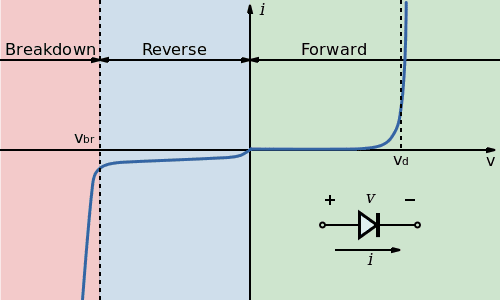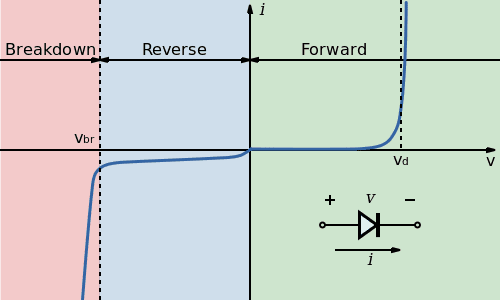# A small thing about diodes

• Sigurdsson

#### Sigurdsson

The following picture represents the current/voltage graph through a basic p-n junction diode.Diodes have a built in potential 0.6 volts. One must overcome this potential to make the diode work. This 0.6V are the nearly flat line in the forward region. Am I right?

My question is:
If you connect a diode in a closed circuit with resistance and a battery (+5V), then would Kirchoff work this way?

0 = 5V - 0.6V - 4.4V

Is the diode simply -0.6V?

And one more thing, what happens if the battery is only +0.5V?

The following picture represents the current/voltage graph through a basic p-n junction diode.Diodes have a built in potential 0.6 volts. One must overcome this potential to make the diode work. This 0.6V are the nearly flat line in the forward region. Am I right?

My question is:
If you connect a diode in a closed circuit with resistance and a battery (+5V), then would Kirchoff work this way?

0 = 5V - 0.6V - 4.4V

Is the diode simply -0.6V?

And one more thing, what happens if the battery is only +0.5V?

You are correct. And if the battery is only supplying 0.5V, then you are still on the right side of the plot before Vd, so very little forward current flows.